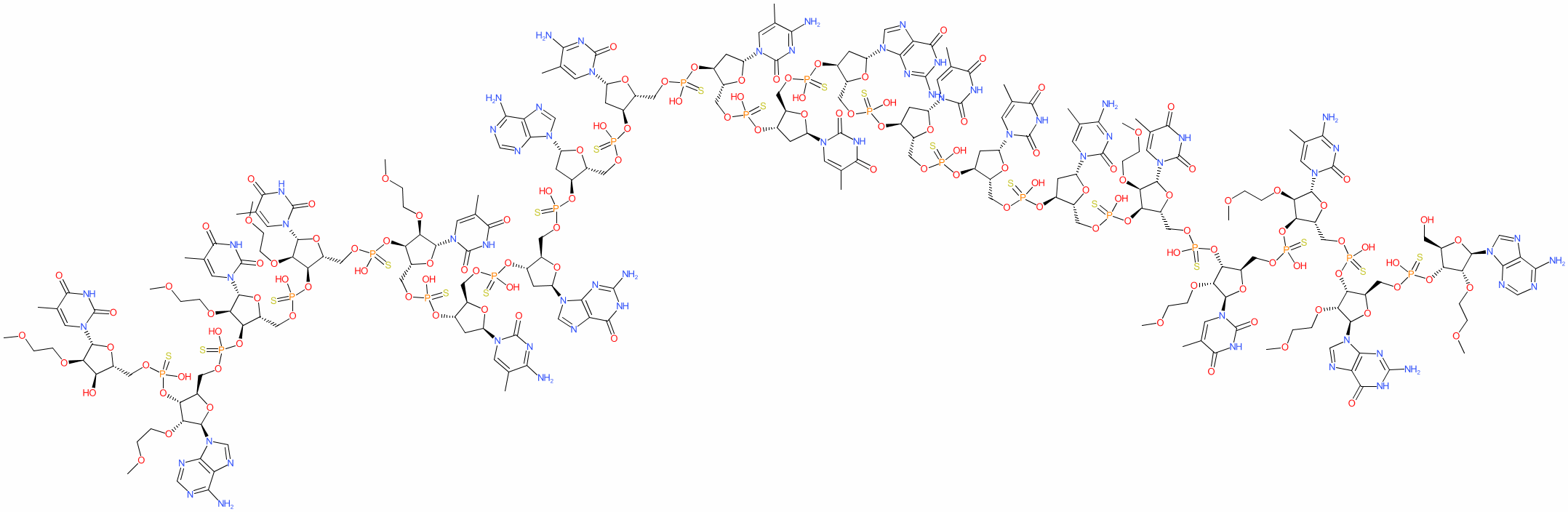#### GtoPdb Ligand ID: 8681

Synonyms: APOC-III antisense inhibitor | ISIS 304801 | ISIS-APOCIIIRx | Waylivra®
volanesorsen is an approved drug (EMA (2019))
Compound class: Synthetic organic
Comment: Volanesorsen is an antisense oligonucleotide (ASO) designed to reduce levels of apolipoprotein C-III (APOC3) in patients with elevated blood triglyceride levels . Reducing triglycerides via APOC3 could be beneficial in patients with inherited hypertriglyceridemia (e.g. those with familial chylomicronemia syndrome, FCS) . The role of APOC3 in hypertriglyceridemia and the effects APOC3 ASO use are reviewed in .
HELM annotation for this RNA (from PubChem) is RNA1{[MOE](A).[sp][MOE](G).[sp][MOE]([5meC]).[sp][MOE](T).[sp][MOE](T).[sp][dR]([5meC]).[sp][dR](T).[sp][dR](T).[sp][dR](G).[sp][dR](T).[sp][dR]([5meC]).[sp][dR]([5meC]).[sp][dR](A).[sp][dR](G).[sp][dR]([5meC]).[sp][MOE](T).[sp][MOE](T).[sp][MOE](T).[sp][MOE](A).[sp][MOE](T)}\$\$\$\$.
2D StructurePhysico-chemical PropertiesHydrogen bond acceptors 130 Hydrogen bond donors 44 Rotatable bonds 156 Topological polar surface area 3216.79 Molecular weight 7160.03 XLogP 6.58 No. Lipinski's rules broken 4
SMILES / InChI / InChIKeyCanonical SMILES COCCO[C@@H]1[C@H](OP(=S)(OC[C@H]2O[C@H](C[C@@H]2OP(=S)(OC[C@H]2O[C@H](C[C@@H]2OP(=S)(OC[C@H]2O[C@H](C[C@@H]2OP(=S)(OC[C@H]2O[C@H](C[C@@H]2OP(=S)(OC[C@H]2O[C@H](C[C@@H]2OP(=S)(OC[C@H]2O[C@H](C[C@@H]2OP(=S)(OC[C@H]2O[C@H](C[C@@H]2OP(=S)(OC[C@H]2O[C@H](C[C@@H]2OP(=S)(OC[C@H]2O[C@H](C[C@@H]2OP(=S)(OC[C@H]2O[C@H](C[C@@H]2OP(=S)(OC[C@H]2O[C@H]([C@@H]([C@@H]2OP(=S)(OC[C@H]2O[C@H]([C@@H]([C@@H]2OP(=S)(OC[C@H]2O[C@H]([C@@H]([C@@H]2OP(=S)(OC[C@H]2O[C@H]([C@@H]([C@@H]2OP(=S)(OC[C@H]2O[C@H]([C@@H]([C@@H]2O)OCCOC)n2cc(C)c(=O)[nH]c2=O)O)OCCOC)n2cnc3c2ncnc3N)O)OCCOC)n2cc(C)c(=O)[nH]c2=O)O)OCCOC)n2cc(C)c(=O)[nH]c2=O)O)OCCOC)n2cc(C)c(=O)[nH]c2=O)O)n2cc(C)c(nc2=O)N)O)n2cnc3c2nc(N)[nH]c3=O)O)n2cnc3c2ncnc3N)O)n2cc(C)c(nc2=O)N)O)n2cc(C)c(nc2=O)N)O)n2cc(C)c(=O)[nH]c2=O)O)n2cnc3c2nc(N)[nH]c3=O)O)n2cc(C)c(=O)[nH]c2=O)O)n2cc(C)c(=O)[nH]c2=O)O)n2cc(C)c(nc2=O)N)O)[C@H](O[C@H]1n1cc(C)c(=O)[nH]c1=O)COP(=S)(O[C@@H]1[C@@H](COP(=S)(O[C@@H]2[C@@H](COP(=S)(O[C@@H]3[C@@H](COP(=S)(O[C@@H]4[C@@H](CO)O[C@H]([C@@H]4OCCOC)n4cnc5c4ncnc5N)O)O[C@H]([C@@H]3OCCOC)n3cnc4c3nc(N)[nH]c4=O)O)O[C@H]([C@@H]2OCCOC)n2cc(C)c(nc2=O)N)O)O[C@H]([C@@H]1OCCOC)n1cc(C)c(=O)[nH]c1=O)O Isomeric SMILES COCCO[C@@H]1[C@H](OP(=S)(OC[C@H]2O[C@H](C[C@@H]2OP(=S)(OC[C@H]2O[C@H](C[C@@H]2OP(=S)(OC[C@H]2O[C@H](C[C@@H]2OP(=S)(OC[C@H]2O[C@H](C[C@@H]2OP(=S)(OC[C@H]2O[C@H](C[C@@H]2OP(=S)(OC[C@H]2O[C@H](C[C@@H]2OP(=S)(OC[C@H]2O[C@H](C[C@@H]2OP(=S)(OC[C@H]2O[C@H](C[C@@H]2OP(=S)(OC[C@H]2O[C@H](C[C@@H]2OP(=S)(OC[C@H]2O[C@H](C[C@@H]2OP(=S)(OC[C@H]2O[C@H]([C@@H]([C@@H]2OP(=S)(OC[C@H]2O[C@H]([C@@H]([C@@H]2OP(=S)(OC[C@H]2O[C@H]([C@@H]([C@@H]2OP(=S)(OC[C@H]2O[C@H]([C@@H]([C@@H]2OP(=S)(OC[C@H]2O[C@H]([C@@H]([C@@H]2O)OCCOC)n2cc(C)c(=O)[nH]c2=O)O)OCCOC)n2cnc3c2ncnc3N)O)OCCOC)n2cc(C)c(=O)[nH]c2=O)O)OCCOC)n2cc(C)c(=O)[nH]c2=O)O)OCCOC)n2cc(C)c(=O)[nH]c2=O)O)n2cc(C)c(nc2=O)N)O)n2cnc3c2nc(N)[nH]c3=O)O)n2cnc3c2ncnc3N)O)n2cc(C)c(nc2=O)N)O)n2cc(C)c(nc2=O)N)O)n2cc(C)c(=O)[nH]c2=O)O)n2cnc3c2nc(N)[nH]c3=O)O)n2cc(C)c(=O)[nH]c2=O)O)n2cc(C)c(=O)[nH]c2=O)O)n2cc(C)c(nc2=O)N)O)[C@H](O[C@H]1n1cc(C)c(=O)[nH]c1=O)COP(=S)(O[C@@H]1[C@@H](COP(=S)(O[C@@H]2[C@@H](COP(=S)(O[C@@H]3[C@@H](COP(=S)(O[C@@H]4[C@@H](CO)O[C@H]([C@@H]4OCCOC)n4cnc5c4ncnc5N)O)O[C@H]([C@@H]3OCCOC)n3cnc4c3nc(N)[nH]c4=O)O)O[C@H]([C@@H]2OCCOC)n2cc(C)c(nc2=O)N)O)O[C@H]([C@@H]1OCCOC)n1cc(C)c(=O)[nH]c1=O)O InChI Key IJUQCWMZCMFFJP-GQSLRNSLSA-N
 ClassificationCompound class Synthetic organic Approved drug? Yes (EMA (2019))
 IUPAC NameAde-MeOEt(-2)Ribf-sP-Gua-MeOEt(-2)Ribf-sP-m5Cyt-MeOEt(-2)Ribf-sP-Thy-MeOEt(-2)Ribf-sP-Thy-MeOEt(-2)Ribf-sP-m5Cyt-dRibf-sP-dThd-sP-dThd-sP-dGuo-sP-dThd-sP-m5Cyt-dRibf-sP-m5Cyt-dRibf-sP-dAdo-sP-dGuo-sP-m5Cyt-dRibf-sP-Thy-MeOEt(-2)Ribf-sP-Thy-MeOEt(-2)Ribf-sP-Thy-MeOEt(-2)Ribf-sP-Ade-MeOEt(-2)Ribf-sP-Thy-MeOEt(-2)Ribf
 International Nonproprietary NamesINN number INN 10166 volanesorsen
 SynonymsAPOC-III antisense inhibitor | ISIS 304801 | ISIS-APOCIIIRx | Waylivra®
 Database LinksCAS Registry No. 915430-78-3 (source: WHO INN record) GtoPdb PubChem SID 252166881 PubChem CID 121494123 Search Google for chemical match using the InChIKey IJUQCWMZCMFFJP-GQSLRNSLSA-N Search Google for chemicals with the same backbone IJUQCWMZCMFFJP Search PubMed clinical trials volanesorsen Search PubMed titles volanesorsen Search PubMed titles/abstracts volanesorsen UniChem Compound Search for chemical match using the InChIKey IJUQCWMZCMFFJP-GQSLRNSLSA-N UniChem Connectivity Search for chemical match using the InChIKey IJUQCWMZCMFFJP-GQSLRNSLSA-N